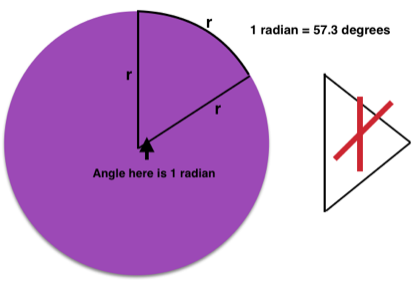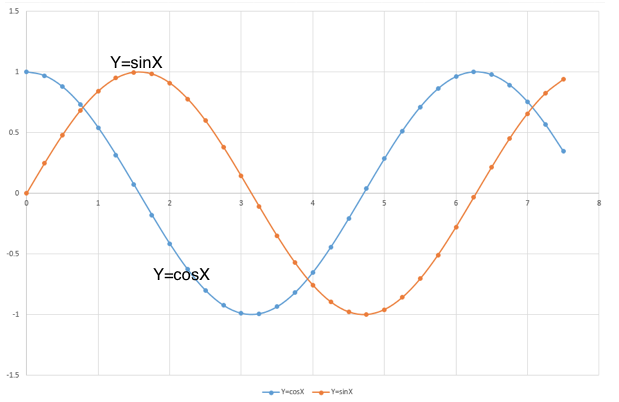### Sine and cosine graphs

#### Measuring angles in radians

In A level physics that is study from 16 years plus, it is common to measure angles in radians rather that degrees. The angles used in the graphs below are measured in radians. A radian is the angle shown in this diagram.Radian explanation

The distance around the edge of the circle in this segment is equal to one radius. The angle marked at the centre is one radian. This is not the same as an equilateral triangle where each angle would be 60 degrees, a little more than one radian.

Since the radius of a circle fits into the circumference 2π times that means there are 2π radians in a complete circle

Since there are 360 degrees in a circle then 2π radians = 360 degrees so one radian =57.3 degrees (approx.)

The sine graph is in yellow and the cosine graph in blue. The shapes are identical. These are very important graphs in the study of waves and in simple harmonic motion.Other pages related to graphs and general physics ideas are: